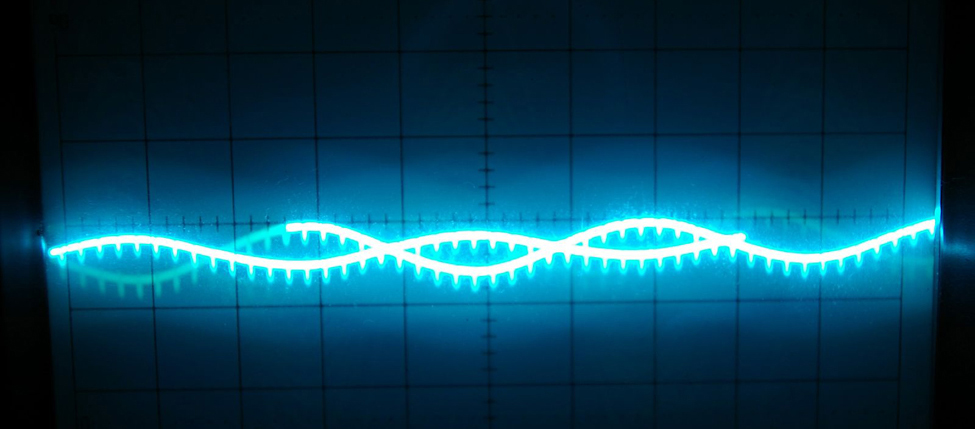## Introduction to Trigonometric Identities and EquationsFigure 1. A sine wave models disturbance. (credit: modification of work by Mikael Altemark, Flickr).

Math is everywhere, even in places we might not immediately recognize. For example, mathematical relationships describe the transmission of images, light, and sound. The sinusoidal graph in (Figure) models music playing on a phone, radio, or computer. Such graphs are described using trigonometric equations and functions. In this chapter, we discuss how to manipulate trigonometric equations algebraically by applying various formulas and trigonometric identities. We will also investigate some of the ways that trigonometric equations are used to model real-life phenomena.